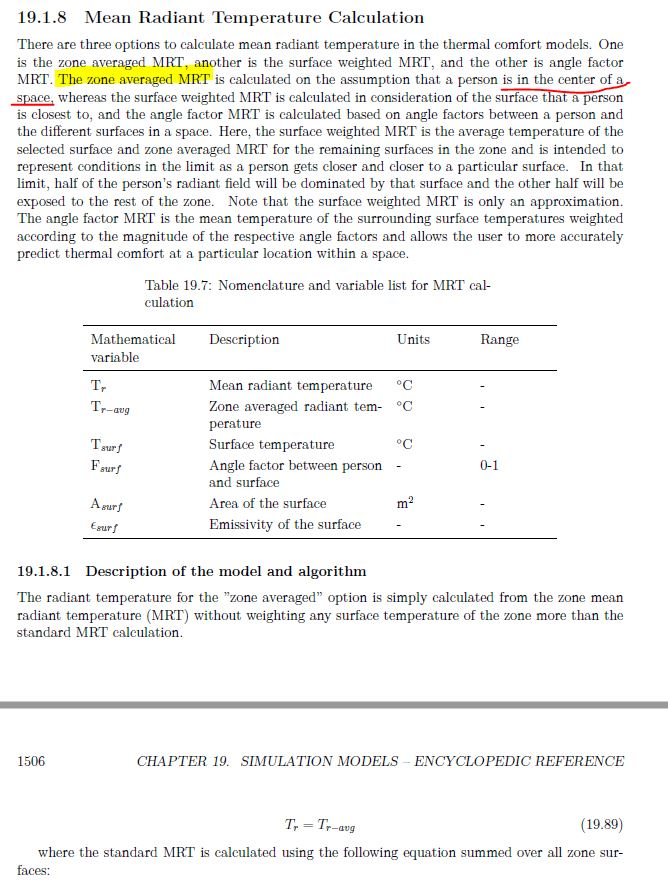Question-and-Answer Resource for the Building Energy Modeling Community
Get started with the Help page

# Radiant temperature - calculation point

Hi all,

I´m looking at comfort conditions in a space with a glazed roof and radiant cooling slab. The Zone is 6m high, so I was wondering which height in the space does the Radiant Temperature Calculation represent.

I had somehow assumed it would be the centroid, so 3m, but looking at the engineering manual it doesn´t seem 100% clear to me. I assume that the Zone averaged MRT is the default method if nothing else is specified.

If the case above is correct, and results are reported for 3m high,

A. Is there a way to get the results for ZoneMeanRadiant/ZoneOperative temperatures at 1.1m high?

The manual refers to the mean radiant calculation in relation to thermal comfort models, but I don´t seem to find its relation to the ZoneMeanRadiantTemperature, ZoneOperativeTemperature reporting variables.

B. Does anyone know where to define any of the three options: zone averaged, surface weighted or angle factor?

Thanks! Rafaeledit retag close merge delete

Does anyone know any lead to look into this? Thanks

Sort by » oldest newest most voted

You are correct that the Zone Averaged MRT is the default algorithm (link to updated Engineering Reference Section). This approach is calculating the MRT based on the surface area, temperature, and emissivity of each zone's surface.

$$T_{zone-averaged} = \Sigma \frac{\epsilon_i * A_i *T_i}{\epsilon_i * A_i }$$

where "i" is for each surface in a zone. This isn't necessarily positioning the occupant in the centroid of the zone -- just calculating a fictitious "average" MRT weighted by areas and emissivity.

A. Yes, you could ask EnergyPlus to calculate MRT for a specific location in a zone using either of the other two options. Surface Weighted MRT is going to calculate an average of the surface temperature that occupants are closest to and the averaged MRT of all other surfaces in the zone.

$$T_{surface-weighted} = \frac{T_{radiant-others} + T_{surface}}{2}$$

where

$$T_{radiant-others} = \Sigma \frac{\epsilon_i * A_i *T_i}{\epsilon_i * A_i }$$

and "i" is for all other surfaces in zone. This is a decent approximation, but if you want to know MRT for a very specific location in the zone, then you should rather use the Angle Factor MRT option. This calculated MRT like the first Zone Averaged MRT option, but weights surfaces by their view factors (VF) to the occupant(s) instead of their surface areas and uses T^4 instead of T.

$$T_{angle-factor} = \Sigma \frac{\epsilon_i * VF_i *T_i^4}{\epsilon_i * VF_i }$$

However, this requires that the user adds a ComfortViewFactorAngles object to the IDF that sets each surface's view factor so you'll have to manually calculate them or have another tool calculate them for you.

No matter which of the three MRT methods you use, the output variable you want to examine is Zone Thermal Comfort Mean Radiant Temperature

B. You define which of the three MRT methods you want to use in the Mean Radiant Temperature Calculation Type input field of the People object assigned to the zone where you want the MRT calculations to take place.

more